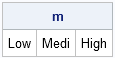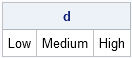When you create a character matrix in SAS/IML software, the initial values determine the number of characters that can fit into any element of the matrix. For example, the following statements define a 1x3 character matrix:

```proc iml; m = {"Low" "Med" "High"};```

After the matrix is defined, at most four characters can fit into any element of m. For example, if you try to replace the second element of the matrix with the word "Medium," only the first four characters are stored:

```m = "Medium"; print m;```There is an easy way to reallocate the character matrix so that it can hold words with more than four characters. The trick is to use the PUTC function in Base SAS software to copy the vector:

```d = putc(m, "\$6."); /** copy into vector with length 6 **/ d = "Medium"; /** value fits without truncation **/ print d;```The PUTC function applies the \$6. format to each element of the SAS/IML vector. Consequently, the d vector can store up to six characters.

There might be times when you don't know how many characters you need for the d vector until the program runs. For example, perhaps you are reading the value "Medium" from a file and you don't know its length until the program runs. No problem. The following statements can use the NLENG function in SAS/IML software to determine the length of characters in "Medium":

```v = "Medium";   /** assume the value of v is not known until the program runs **/ w = nleng(v); fmt = "\$" + strip(char(w)) + "."; /** form the \$w. format **/ d = putc(m, fmt); /** copy into vector with length w **/ d = v; /** value fits without truncation **/```

Notice the use of the powerful STRIP(CHAR(...)) combination, which I described in a previous article. The fmt variable contains the name of the format that is applied by the PUTC function.

This tip is also found in Chapter 2 of my book, Statistical Programming with SAS/IML Software. You can download a PDF of Chapter 2 from my book's Web site.

ShareDistinguished Researcher in Computational Statistics

Rick Wicklin, PhD, is a distinguished researcher in computational statistics at SAS and is a principal developer of PROC IML and SAS/IML Studio. His areas of expertise include computational statistics, simulation, statistical graphics, and modern methods in statistical data analysis. Rick is author of the books Statistical Programming with SAS/IML Software and Simulating Data with SAS.

1.•